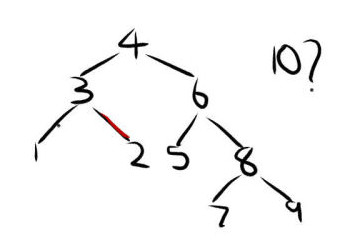# [Solved]: How does insertion work in an AVL tree?Problem Detail:From the above image, while trying to maintain an AVL tree data structure, how would the tree look after inserting the value 10? Also, if anyone has any suggestions or simple method of rotating, feel free to share. I am a bit lost with this idea of maintaining a certain height in this data structure.

AVL trees should be binary search trees. In the example you gave, 2 is the right child of 3. That isn’t right since 2 < 3. Assume the 2 and 3 are switched, which results in a correct binary tree.
Remember the rule for AVL trees: for any node, the height difference between the left child and right child is at most 1. Following the tree down, the 10 is inserted as the right child of 9. This breaks the rule. Look at the subtree rooted at 6. The height of the left child is 0 while the height of the right child is 2. The tree must be rotated, which is the tricky part. 8 becomes the right child of 4. 6 becomes the left child of 8. 7 becomes the right child of 6. After all of that, the final AVL tree would look like this:(courtesy of Uottawa). I always visualized rotations like this:

1. With your left hand, “hold” the 6 and “detach” it from the 4.
2. With your right hand, “hold” the 8.
3. “Drop” the 6 so that it swings counterclockwise, due to gravity of course. (6 is now the left child of 8)
4. The 6 “hits” the 7. (7 is now the right child of 6)
5. “Attach” this whole subtree (now rooted at 8, since the 6 “fell”) back onto the 4.